Documentation

### This is machine translation

Mouseover text to see original. Click the button below to return to the English version of the page.

## Select ARCH Lags for GARCH Model Using Econometric Modeler App

This example shows how to select the appropriate number of ARCH and GARCH lags for a GARCH model by using the Econometric Modeler app. The data set, stored in `Data_MarkPound`, contains daily Deutschmark/British pound bilateral spot exchange rates from 1984 through 1991.

### Import Data into Econometric Modeler

At the command line, load the `Data_MarkPound.mat` data set.

`load Data_MarkPound`

At the command line, open the Econometric Modeler app.

`econometricModeler`

Alternatively, open the app from the apps gallery (see Econometric Modeler).

Import `Data` to the app:

1. On the Econometric Modeler tab, in the Import section, click.

2. In the Import Data dialog box, in the Import? column, select the check box for the Data1 variable.

3. Click .

The variable `Data1` appears in the Data Browser, and its time series plot appears in the Time Series Plot(Data1) figure window.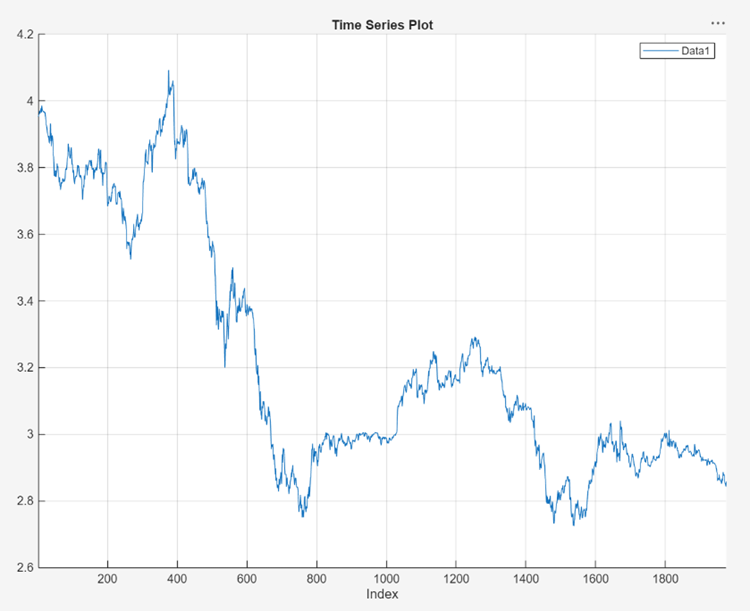The exchange rate looks nonstationary (it does not appear to fluctuate around a fixed level).

### Transform Data

Convert the exchange rates to returns.

1. With `Data1` selected in the Data Browser, on the Econometric Modeler tab, in the Transforms section, click .

In the Data Browser, a variable representing the logged exchange rates (`Data1Log`) appears , and its time series plot appears in the Time Series Plot(Data1Log) figure window.

2. In the Data Browser, select `Data1Log`.

3. On the Econometric Modeler tab, in the Transforms section, click .

In the Data Browser, a variable representing the returns (`Data1LogDiff`) appears. A time series plot of the differenced series appears in the Time Series Plot(Data1LogDiff) figure window.

### Check for Autocorrelation

Rename the `Data1LogDiff` variable to `Returns`.

1. In the Data Browser, right-click `Data1LogDiff`.

2. In the context menu, select Rename.

3. Enter `Returns`.

The app updates the names of all documents associated with the returns.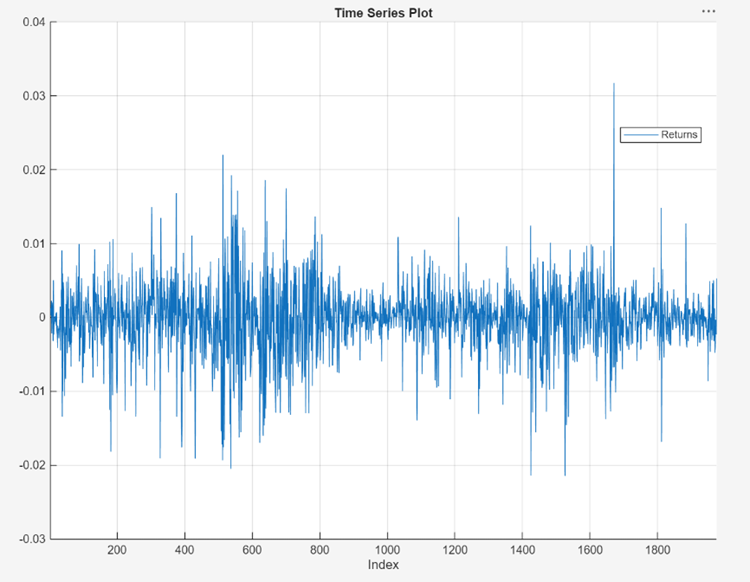The returns series fluctuates around a common level, but exhibits volatility clustering. Large changes in the returns tend to cluster together, and small changes tend to cluster together. That is, the series exhibits conditional heteroscedasticity.

Visually assess whether the returns have serial correlation by plotting the sample ACF and PACF:

1. Close all figure windows in the right pane.

2. In the Data Browser, select the `Returns` time series.

3. Click the Plots tab, then click .

4. Click the Plots tab, then click .

5. Drag the PACF(Returns) figure window below the ACF(Returns) figure window so that you can view them simultaneously.The sample ACF and PACF show virtually no significant autocorrelation.

Conduct the Ljung-Box Q-test to assess whether there is significant serial correlation in the returns for at most 5, 10, and 15 lags. To maintain a false-discovery rate of approximately 0.05, specify a significance level of 0.05/3 = 0.0167 for each test.

1. Close the ACF(Returns) and PACF(Returns) figure windows.

2. With `Returns` selected in the Data Browser, on the Econometric Modeler tab, in the Tests section, click > Ljung-Box Q-Test.

3. On the LBQ tab, in the Parameters section, set Number of Lags to `5`.

4. Set Significance Level to `0.0167`.

5. In the Tests section, click Run Test.

6. Repeat steps 3 through 5 twice, with these changes.

1. Set Number of Lags to 10 and the DOF to 10.

2. Set Number of Lags to 15 and the DOF to 15.

The test results appear in the Results table of the LBQ(Returns) document.The Ljung-Box Q-test null hypothesis that all autocorrelations up to the tested lags are zero is not rejected for tests at lags 5, 10, and 15. These results, and the ACF and PACF, suggest that a conditional mean model is not needed for this returns series.

### Check for Conditional Heteroscedasticity

To check the returns for conditional heteroscedasticity, Econometric Modeler requires a series of squared residuals. After importing the squared residuals into the app, visually assess whether there is conditional heteroscedasticity by plotting the ACF and PACF of the squared residuals. Then, determine the appropriate number of lags for a GARCH model of the returns by conducting Engle's ARCH test.

Compute the series of squared residuals at the command line by demeaning the returns, then squaring each element of the result.

Export `Returns` to the command line:

1. In the Data Browser, right-click `Returns`.

2. In the context menu, select `Export`.

`Returns` appears in the MATLAB® Workspace.

Remove the mean from the returns, then square each element of the result. To ensure all series in the Data Browser are synchronized, Econometric Modeler prepends first-differenced series with a `NaN` value. Therefore, to estimate the sample mean, use `nanmean`.

```Residuals = Returns - nanmean(Returns); Residuals2 = Residuals.^2; ```

Create a table containing the `Returns` and `Residuals2` variables.

`Tbl = table(Returns,Residuals,Residuals2);`

Import `Tbl` into Econometric Modeler:

1. On the Econometric Modeler tab, in the Import section, click.

2. The app must clear the Data Browser and all documents before importing new data. Therefore, after clicking , in the Econometric Modeler dialog box, click .

3. In the Import Data dialog box, in the Import? column, select the check box for the Tbl variable.

4. Click .

The variables appear in the Data Browser, and a time series plot of all the series appears in the Time Series Plot(Residuals) figure window.

Plot the ACF and PACF of the squared residuals.

1. Close the Time Series Plot(Residuals) figure window.

2. In the Data Browser, select the `Residuals2` time series.

3. Click the Plots tab, then click .

4. Click the Plots tab, then click .

5. Drag the PACF(Residuals2) figure window below the ACF(Residuals2) figure window so that you can view them simultaneously.The sample ACF and PACF of the squared returns show significant autocorrelation. This result suggests that a GARCH model with lagged variances and lagged squared innovations might be appropriate for modeling the returns.

Conduct Engle's ARCH test on the residuals series. Specify a two-lag ARCH model alternative hypothesis.

1. Close all figure windows.

2. In the Data Browser, select the `Residuals` time series.

3. On the Econometric Modeler tab, in the Tests section, click > Engle's ARCH Test.

4. On the ARCH tab, in the Parameters section, set Number of Lags to `2`.

5. In the Tests section, click .

The test results appear in the Results table of the ARCH(Residuals) document.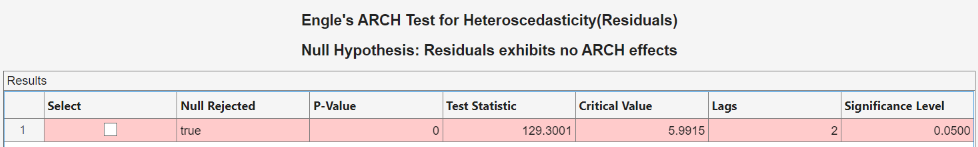Engle's ARCH test rejects the null hypothesis of no ARCH effects in favor of the alternative ARCH model with two lagged squared innovations. An ARCH model with two lagged innovations is locally equivalent to a GARCH(1,1) model.

### Create and Fit GARCH Model

Fit a GARCH(1,1) model to the returns series.

1. In the Data Browser, select the `Returns` time series.

2. Click the Econometric Modeler tab. Then, in the Models section, click the arrow to display the models gallery.

3. In the models gallery, in the GARCH Models section, click GARCH.

4. In the GARCH Model Parameters dialog box, on the Lag Order tab:

1. Set GARCH Degree to `1`.

2. Set ARCH Degree to `1`.

3. Because the returns required demeaning, include an offset by selecting the Include Offset check box.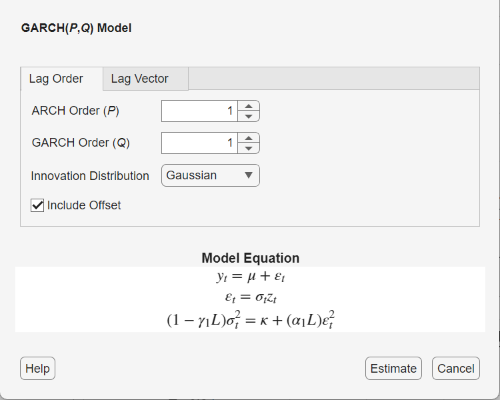5. Click .

The model variable `GARCH_Returns` appears in the Models section of the Data Browser, and its estimation summary appears in the Model Summary(GARCH_Returns) document.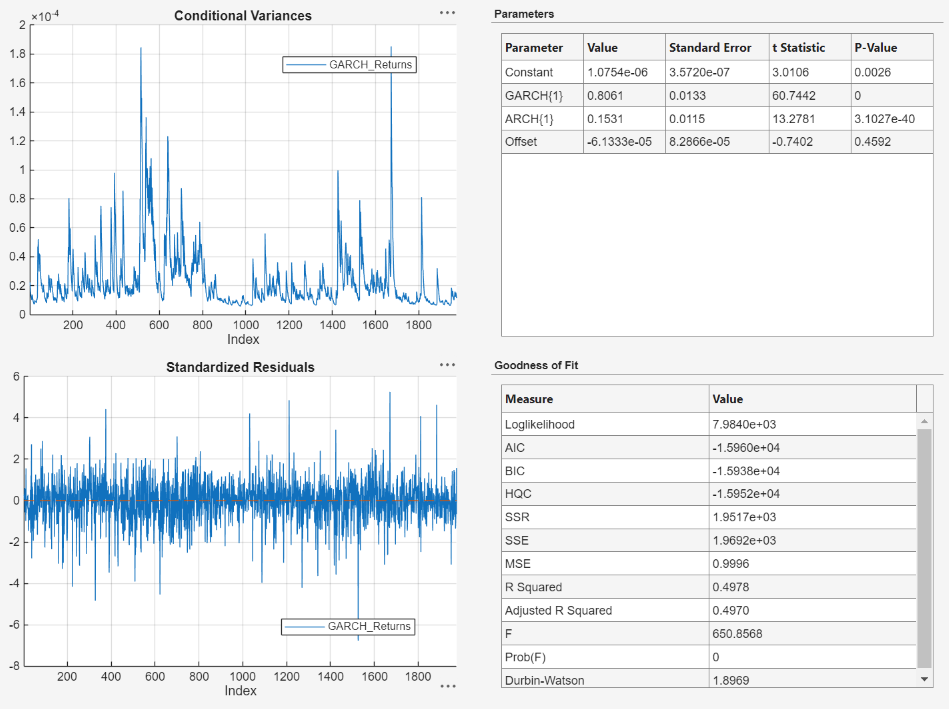An alternative way to select lags for a GARCH model is by fitting several models containing different lag polynomial degrees. Then, choose the model yielding the minimal AIC.